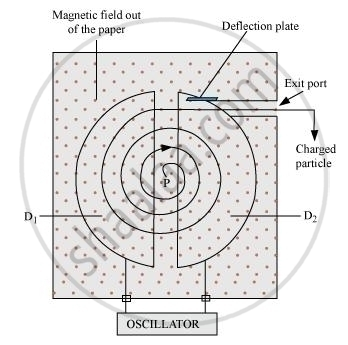Department of Pre-University Education, KarnatakaPUC Karnataka Science Class 12
Advertisement Remove all ads

# Explain the Principle and Working of a Cyclotron with the Help of a Schematic Diagram. Write the Expression for Cyclotron Frequency. - Physics

Advertisement Remove all ads
Advertisement Remove all ads
Advertisement Remove all ads

Explain the principle and working of a cyclotron with the help of a schematic diagram. Write the expression for cyclotron frequency.

Advertisement Remove all ads

#### SolutionCyclotron is a machine used to accelerate charged particles or ions to high energies. It uses both electrical and magnetic fields in combination to increase the speed of the charged particles.

The particles move in two semi-circular containers D1 and D, called Dees. Inside the metal box, the charged particle is shielded from external electric fields.

When the particle moves from one dee to another, electric field is acted on the particle.

The sign of the electric field is changed alternately, in tune with the circular motion of the particle. Hence, the particle is always accelerated by the electric field. As the energy of the particle increases, the radius of the circular path increases.

qcB = (mv_2)/r

r= (mv)/(qB)

Time taken for a particle for one complete revolution = T

T  = (2pir)/v

T =(2pim)/(qB)

T = 1/v_c

Where v_cis the cyclotron frequency

Then,

I/v_c = (2pim)/(qB)

v_c = (qB)/(2pim)

The above expression is the expression for cyclotron frequency.

The oscillator applies an ac voltage across the Ds and this voltage must have a frequency equal to that of cyclotron frequency.

Concept: Motion in Combined Electric and Magnetic Fields - Cyclotron
Is there an error in this question or solution?

#### Video TutorialsVIEW ALL 

Advertisement Remove all ads
Share
Notifications

View all notifications

Forgot password?